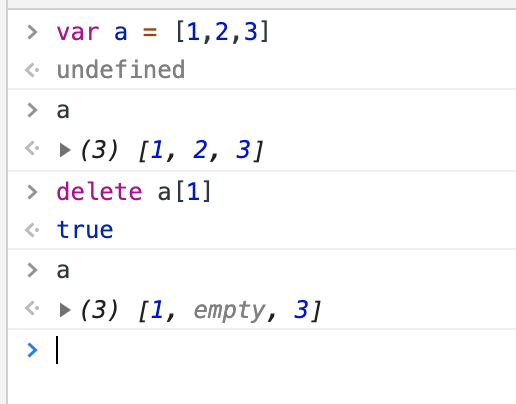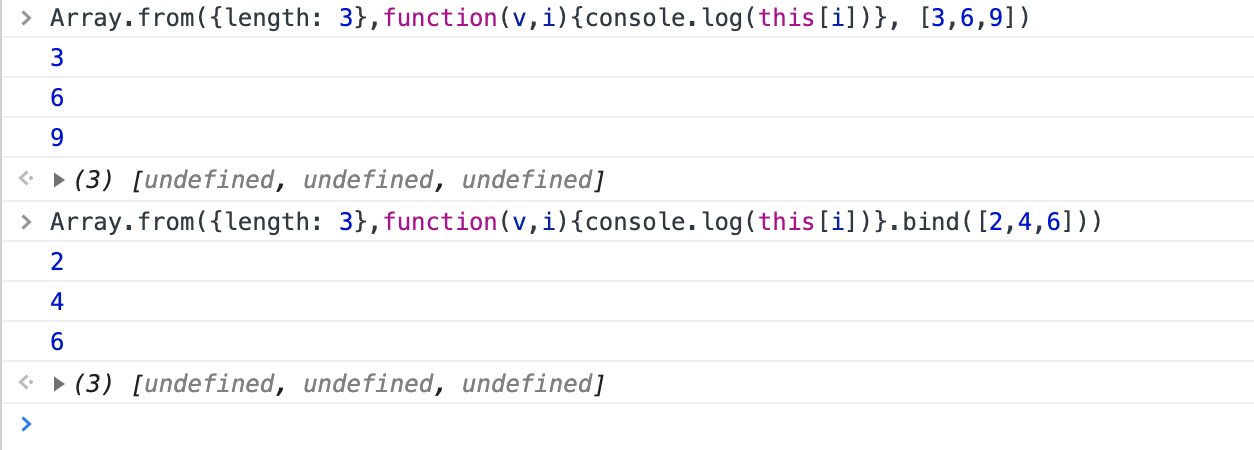# 温故知新： Array

## 1. `delete` 数组成员## 2. `Array.from(arrayLike[, mapFn[, thisArg]])`

`Array.from` 有参数二 `mapFn` ，存在时则新数组中的每个元素都会执行该回调。

`Array.from` 参数三 是 `thisArg`, 在`mapFn`执行时的`this`对象。效果和bind一样。`Array.from` 将类数组转为数组， 也可以用扩展运算符 `[...args]` 将类数组转为数组。 ES5中是可以用 `Array.prototype.slice`将类数组转为数组的

## `Array.isArray`

`Array.isArray(Array.prototype); ` 记住：数组的原型也是一个数组

instanceof 不能检测 iframes

``````xArray = window.frames[window.frames.length-1].Array;
var arr = new xArray(1,2,3); // [1,2,3]
Array.isArray(arr);  // true
arr instanceof Array; // false
``````

## `Array.of`

Array.of() 方法创建一个具有可变数量参数的新数组实例，而不考虑参数的数量或类型。

Array.of() 和 Array 构造函数之间的区别在于处理整数参数

``````Array.of(7);       // 
Array.of(1, 2, 3); // [1, 2, 3]

Array(7);          // [ , , , , , , ]
Array(1, 2, 3);    // [1, 2, 3]
``````

## `Array.prototype.copyWithin()`

``````let numbers = [1, 2, 3, 4, 5];

numbers.copyWithin(-2);
// [1, 2, 3, 1, 2]  将自身复制，复制到倒数第二位开始。

numbers.copyWithin(0, 3);
// [4, 5, 3, 4, 5] 从第三位开始复制，复制到0开始。即 '4,5'复制后，粘贴到0开始。由于'4,5'是两位，所以'1,2'被替换了。
``````

## `Array.prototype.entries()`

``````var array1 = ['a', 'b', 'c'];

var iterator1 = array1.entries();

console.log(iterator1.next().value);
// expected output: Array [0, "a"]

console.log(iterator1.next().value);
// expected output: Array [1, "b"]

``````

## `Array.prototype.flat()`

depth 指定要提取嵌套数组的结构深度，默认值为 1。

``````var arr1 = [1, 2, [3, 4]];
arr1.flat();
// [1, 2, 3, 4]

var arr2 = [1, 2, [3, 4, [5, 6]]];
arr2.flat();
// [1, 2, 3, 4, [5, 6]]

var arr3 = [1, 2, [3, 4, [5, 6]]];
arr3.flat(2);
// [1, 2, 3, 4, 5, 6]

//使用 Infinity 作为深度，展开任意深度的嵌套数组
arr3.flat(Infinity);
// [1, 2, 3, 4, 5, 6]
``````

## `Array.prototype.flatMap()`

``````var new_array = arr.flatMap(function callback(currentValue[, index[, array]]) {
// 返回新数组的元素
}[, thisArg])
``````

``````var arr1 = [1, 2, 3, 4];

arr1.map(x => [x * 2]);
// [, , , ]

arr1.flatMap(x => [x * 2]);
// [2, 4, 6, 8]

// 只会将 flatMap 中的函数返回的数组 “压平” 一层
arr1.flatMap(x => [[x * 2]]);
// [, , , ]
``````

## `Array.prototype.keys()`

``````var array1 = ['a', 'b', 'c'];
var iterator = array1.keys();
iterator.next() // {value: 0, done: false}
[...iterator] // [0, 1, 2]
``````

## `Array.prototype.map()`

``````var new_array = arr.map(function callback(currentValue[, index[, array]]) {
// Return element for new_array
}[, thisArg])
``````

## `Array.prototype.reduce()`

callback接收四个参数:

• accumulator: 累计器累计回调的返回值; 它是上一次调用回调时返回的累积值，或initialValue（见于下方）
• currentValue: 数组中正在处理的元素
• currentIndex: (可选)数组中正在处理的当前元素的索引。 如果提供了initialValue，则起始索引号为0，否则为1。
• array: (可选) 调用reduce()的数组

initialValue : 作为第一次调用 callback函数时的第一个参数的值。 如果没有提供初始值，则将使用数组中的第一个元素。 在没有初始值的空数组上调用 reduce 将报错。

``````[1,2,3,4].reduce((a,b) => a + 2 * b)
// 19 由于没有提供初始 initialValue，直接将1作为第一次执行的a。 1 + 2*2 + 2*3 + 2*4
[1,2,3,4].reduce((a,b) => a + 2 * b, 0)
// 20 提供了初始值0  0 + 2*1 + 2*2 + 2*3 + 2*4
``````

## `Array.prototype.reverse()`

reverse() 方法将数组中元素的位置颠倒,并返回该数组。该方法会改变原数组。

## `Array.prototype.slice()`

``````arr.slice();
// [0, end]

arr.slice(begin);
// [begin, end]

arr.slice(begin, end);
// [begin, end)
``````

slice 方法可以用来将一个类数组（Array-like）对象/集合转换成一个新数组。你只需将该方法绑定到这个对象上。 一个函数中的 arguments 就是一个类数组对象的例子。

``````function list() {
return Array.prototype.slice.call(arguments);
}

var list1 = list(1, 2, 3); // [1, 2, 3]
``````

## `Array.prototype.sort()`

• 如果 compareFunction(a, b) 小于 0 ，那么 a 会被排列到 b 之前；
• 如果 compareFunction(a, b) 等于 0 ， a 和 b 的相对位置不变。备注： ECMAScript 标准并不保证这一行为，而且也不是所有浏览器都会遵守（例如 Mozilla 在 2003 年之前的版本）；
• 如果 compareFunction(a, b) 大于 0 ， b 会被排列到 a 之前。

## `Array.prototype.splice()`

``````var myFish = ["angel", "clown", "mandarin", "sturgeon"];
var removed = myFish.splice(2, 0, "drum");

// 运算后的 myFish: ["angel", "clown", "drum", "mandarin", "sturgeon"]
// 被删除的元素: [], 没有元素被删除

var myFish = ['angel', 'clown', 'drum', 'sturgeon'];
var removed = myFish.splice(2, 1, "trumpet");

// 运算后的 myFish: ["angel", "clown", "trumpet", "sturgeon"]
// 被删除的元素: ["drum"]
``````

## `Array.prototype.toLocaleString()`

``````var array1 = [1, 'a', new Date('21 Dec 1997 14:12:00 UTC')];
var localeString = array1.toLocaleString('en', {timeZone: "UTC"});

console.log(localeString);
// expected output: "1,a,12/21/1997, 2:12:00 PM",
// This assumes "en" locale and UTC timezone - your results may vary
``````

## `Array.prototype.toString()`

``````var array1 = [1, 2, 'a', '1a'];

console.log(array1.toString());
// expected output: "1,2,a,1a"

``````

## `Array.prototype.values()`

``````let arr = ['w', 'y', 'k', 'o', 'p'];
let eArr = arr.values();
console.log(eArr.next().value); // w
console.log(eArr.next().value); // y
``````
Written on July 6, 2019